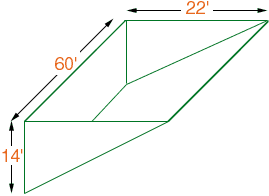Dear sir, My name is Rhett and my problem is...I am a contractor and I am having problems determining the amount of gravel(in tonnage) needed to fill this hole,the measurements are 60' in length,22' in width,and 14' in height....the problem is that it is not a usual box shaped hole or in this case a rectangle shaped hole,but more of a triangular shaped hole.I refer to the triangular shape as the interior shape of the crater not the exterior,which is shaped like the above dimensions.If you could help it would be greatly appreciated.P.s.This was a retaining wall project,built by myself, in front of a sloped end of a yard,so if you could imagine a 14" high hill with a landslide looking face,then build in your mind a three sided rectangle in front of it using the hill side that slopes as the fourth side of the rectangle,then you may be able to imagine what I mean as a triangular shaped interior of the hole. Thank you very much, Rhett Hi Rhett, If I understand correctly this is a diagram of the hole.If this is the correct diagram then you can calculate the volume as the area of the triangular end times the length of 60 feet. The area of a triangle is one-half the base times the height, so you get Volume = 1/2x 2 2 x 14 x 60 = 9240 cubic feet. If you prefer the volume in cubic yards this is  9240/27 = 342.2 cubic yards. Is this the correct diagram? Penny Go to Math Central